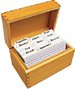# Section 6.5. Combining Pixels: Blurring

[Page 194 (continued)]

### 6.5. Combining Pixels: Blurring

When we make pictures larger (scaling them up), we usually get rough edges: Sharp steps to lines, which we call pixelation. We can reduce pixelation by blurring the image. What we do is set each pixel to an average of pixels around it. In this example, we go through all pixels (note the large loop that surrounds everything) and then in the X and Y dimensions, compute the average of the pixels to either side of the pixel. It takes a picture, and a number (size) of pixels to compute the average.

Of course we need to be careful not to try and access pixels beyond the allowed values of the two-dimensional array of pixels. Try this in the interactions pane:

`> String fileName = FileChooser.getMediaPath("caterpillar.jpg"); > Picture p = new Picture(fileName); [Page 195]> System.out.println(p.getWidth()); 329 > System.out.println(p.getHeight()); 150 > p.getPixel(330,160); java.lang.ArrayIndexOutOfBoundsException: Coordinate out of bounds!   at sun.awt.image.ByteInterleavedRaster.getDataElements     (Unknown Source)   at java.awt.image.BufferedImage.getRGB(Unknown Source)   at SimplePicture.getBasicPixel(SimplePicture.java:247)   at Pixel.setValuesFromPictureAndLocation(Pixel.java:137)   at Pixel.<init>(Pixel.java:57)   at SimplePicture.getPixel(SimplePicture.java:270)   at sun.reflect.NativeMethodAccessorImpl.invoke0(Native Method)   at sun.reflect.NativeMethodAccessorImpl.invoke(Unknown Source)   at sun.reflect.DelegatingMethodAccessorImpl.invoke     (Unknown Source)   at java.lang.reflect.Method.invoke(Unknown Source)`

The java.lang.ArrayIndexOutOfBoundsException tells us that we tried to access an array element that was outside the allowed indices. If this happens when our program is executing, the program will stop and report the exception. This is called a run-time exception because it happens when the program is running rather than at compile time.

So how do we check that the index values are acceptable? We know that the x indices range from 0 to width 1 and the y indices range from 0 to height 1. So we can use

`x >= 0 && x < this.getWidth() && y >= 0 && y < this.getHeight()).`

##### Program 42. A Simple Blur (This item is displayed on pages 195 - 196 in the print version)`/** * Method to blur the pixels * @param numPixels the number of pixels to average in all * directions so if the numPixels is 2 then we will average * all pixels in the rectangle defined by 2 before the * current pixel to 2 after the current pixel */ public void blur(int numPixels) { Pixel pixel = null; Pixel samplePixel = null; int redValue = 0; int greenValue = 0; int blueValue = 0; int count = 0; [Page 196] // loop through the pixels for (int x=0; x < this.getWidth(); x++) { for (int y=0; y < this.getHeight(); y++) { // get the current pixel pixel = this.getPixel(x,y); // reset the count and red, green, and blue values count = 0; redValue = greenValue = blueValue = 0; /* loop through pixel numPixels before x to * numPixels after x */ for (int xSample = x - numPixels; xSample <= x + numPixels; xSample++) { for (int ySample = y - numPixels; ySample <= y + numPixels; ySample++) { /* check that we are in the range of acceptable * pixels */ if (xSample >= 0 && xSample < this.getWidth() && ySample >= 0 && ySample < this.getHeight()) { samplePixel = this.getPixel(xSample,ySample); redValue = redValue + samplePixel.getRed(); greenValue = greenValue + samplePixel.getGreen(); blueValue = blueValue + samplePixel.getBlue(); count = count + 1; } } } // use average color of surrounding pixels Color newColor = new Color(redValue / count, greenValue / count, blueValue / count); pixel.setColor(newColor); } } }`

Here is how to use this method:

`> Picture p = new Picture(     FileChooser.getMediaPath("flower1.jpg")); > p = p.scaleUp(2); > p.explore(); > p.blur(2); > p.explore();`

[Page 197]

Figure 6.13 shows the flower from the collage made bigger, then blurred. You can see the pixelation in the bigger versionthe sharp, blocky edges. With the blur, some of that pixelation goes away. More careful blurs take into account regions of colors (so that edges between colors are kept sharp), and thus are able to reduce pixelation without removing sharpness.

##### Figure 6.13. Making the flower bigger, then blurring to reduce pixelation.Introduction to Computing & Programming Algebra in Java(c) A Multimedia Approach
ISBN: N/A
EAN: N/A
Year: 2007
Pages: 191

flylib.com © 2008-2017.
If you may any questions please contact us: flylib@qtcs.net